# 不同经营目标下考虑质量水平的供应链定价博弈研究Research on Supply Chain Pricing Game Considering Quality Level under Different Business Objectives

DOI: 10.12677/ORF.2018.83014, PDF, HTML, XML, 下载: 626  浏览: 1,539

Abstract: In order to solve the pricing problem of two-level supply chain composed of two competitive man-ufacturers and one retailer, the influence of quality level on pricing decision under different strategies is studied, and the pricing decision model of supply chain under different business ob-jectives is constructed. The optimal pricing and optimal profit of the model are obtained by Stackelberg game, and the impact of quality level on wholesale price and retail price is numerically simulated. The results show that the wholesale price and retail price under the strategy of its own profit are higher than the wholesale price and retail price under the strategy of relative profit. Under the two strategies, two manufacturers’ profit is higher under the strategy of their own profit and the retailer's profit under the strategy of relative profit is higher.

1. 引言

2. 问题描述及符号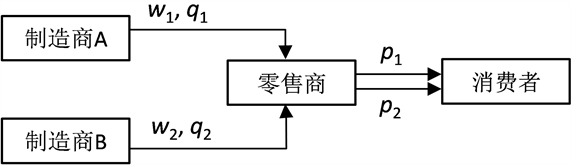Figure 1. Competitive supply chain

${d}_{1}={a}_{1}-{\beta }_{1}{p}_{1}+{b}_{1}{q}_{1}+{\gamma }_{1}{p}_{2}-{b}_{0}{q}_{2}$ (1)

${d}_{2}={a}_{2}-{\beta }_{2}{p}_{2}+{b}_{2}{q}_{2}+{\gamma }_{1}{p}_{1}-{b}_{0}{q}_{1}$ (2)

${\pi }_{m1}={w}_{1}{d}_{1}-\frac{1}{2}{k}_{1}{q}_{1}^{2}$ (3)

${\pi }_{m2}={w}_{2}{d}_{2}-\frac{1}{2}{k}_{2}{q}_{2}^{2}$ (4)

${\pi }_{R}=\left({p}_{1}-{w}_{1}\right){d}_{1}+\left({p}_{2}-{w}_{2}\right){d}_{2}$ (5)

3. 模型求解与分析

3.1. 以自身利润为经营目标

${\pi }_{R}$ 的Hessian矩阵为

$H=\left[\begin{array}{cc}\frac{{\partial }^{2}{\pi }_{R}}{\partial {p}_{1}^{2}}& \frac{{\partial }^{2}{\pi }_{R}}{\partial {p}_{1}\partial {p}_{2}}\\ \frac{{\partial }^{2}{\pi }_{R}}{\partial {p}_{2}\partial {p}_{1}}& \frac{{\partial }^{2}{\pi }_{R}}{\partial {p}_{2}^{2}}\end{array}\right]=\left[\begin{array}{cc}-2{\beta }_{1}& 2{\gamma }_{1}\\ 2{\gamma }_{1}& -2{\beta }_{2}\end{array}\right]$ (6)

${\pi }_{R}$ 关于 ${p}_{1},{p}_{2}$ 的一阶偏导数

$\begin{array}{l}\frac{\partial {\pi }_{R}}{\partial {p}_{1}}=A-2{p}_{1}{\beta }_{1}+2{p}_{2}{\gamma }_{1}+{w}_{1}{\beta }_{1}-{w}_{2}{\gamma }_{1}\\ \frac{\partial {\pi }_{R}}{\partial {p}_{2}}=B-2{p}_{2}{\beta }_{2}+2{p}_{1}{\gamma }_{1}+{w}_{2}{\beta }_{2}-{w}_{1}{\gamma }_{1}\end{array}$

${p}_{1}^{o}=\frac{{\beta }_{2}\left(A+{w}_{1}{\beta }_{1}\right)+{\gamma }_{1}\left(B-{w}_{1}{\gamma }_{1}\right)}{2\left({\beta }_{1}{\beta }_{2}-{\gamma }_{1}^{2}\right)}$ (7)

${p}_{2}^{o}=\frac{{\beta }_{1}\left(B+{w}_{2}{\beta }_{2}\right)+{\gamma }_{1}\left(A-{w}_{2}{\gamma }_{1}\right)}{2\left({\beta }_{1}{\beta }_{2}-{\gamma }_{1}^{2}\right)}$ (8)

${p}_{1}^{o}$${p}_{2}^{o}$ 代入(3)，(4)，求解

$\left\{\begin{array}{l}\frac{\partial {\pi }_{m}{}_{1}}{\partial {w}_{1}}=\frac{1}{2}\left(A-2{w}_{1}{\beta }_{1}+{w}_{2}{\gamma }_{1}\right)=0\\ \frac{\partial {\pi }_{m}{}_{2}}{\partial {w}_{2}}=\frac{1}{2}\left(B-2{w}_{2}{\beta }_{2}+{w}_{1}{\gamma }_{1}\right)=0\end{array}$

${w}_{1}^{o}=\frac{2{\beta }_{2}A+{\gamma }_{1}B}{4{\beta }_{1}{\beta }_{2}-{\gamma }_{1}^{2}}$ (9)

${w}_{2}^{o}=\frac{2{\beta }_{1}B+{\gamma }_{1}A}{4{\beta }_{1}{\beta }_{2}-{\gamma }_{1}^{2}}$ (10)

${p}_{1}^{o}=\frac{\left(6{\beta }_{1}{\beta }_{2}-3{\gamma }_{1}^{2}\right){\beta }_{2}A+\left(5{\beta }_{1}{\beta }_{2}-2{\gamma }_{1}^{2}\right){\gamma }_{1}B}{2\left({\beta }_{1}{\beta }_{2}-{\gamma }_{1}^{2}\right)\left(4{\beta }_{1}{\beta }_{2}-{\gamma }_{1}^{2}\right)}$

${p}_{2}^{o}=\frac{\left(5{\beta }_{1}{\beta }_{2}-2{\gamma }_{1}^{2}\right){\gamma }_{1}A+\left(6{\beta }_{1}{\beta }_{2}-3{\gamma }_{1}^{2}\right){\beta }_{1}B}{2\left({\beta }_{1}{\beta }_{2}-{\gamma }_{1}^{2}\right)\left(4{\beta }_{1}{\beta }_{2}-{\gamma }_{1}^{2}\right)}$

${p}_{1}^{o}$${p}_{2}^{o}$${w}_{1}^{o}$${w}_{2}^{o}$ 代入(3)、(4)、(5)可得制造商最优利润、零售商最优利润：

${\pi }_{m1}^{o}=\frac{{\beta }_{1}{\left({\gamma }_{1}B+2{\beta }_{2}A\right)}^{2}-{k}_{1}{q}_{1}^{2}{\left(4{\beta }_{1}{\beta }_{2}-{\gamma }_{1}^{2}\right)}^{2}}{2{\left(4{\beta }_{1}{\beta }_{2}-{\gamma }_{1}^{2}\right)}^{2}},$

${\pi }_{m2}^{o}=\frac{{\beta }_{2}{\left({\gamma }_{1}A+2{\beta }_{1}B\right)}^{2}-{k}_{2}{q}_{2}^{2}{\left(4{\beta }_{1}{\beta }_{2}-{\gamma }_{1}^{2}\right)}^{2}}{2{\left(4{\beta }_{1}{\beta }_{2}-{\gamma }_{1}^{2}\right)}^{2}},$

${\pi }_{R}^{o}=\frac{\left(4{\beta }_{1}{\beta }_{2}+5{\gamma }_{1}^{2}\right)\left({\beta }_{2}{A}^{2}+{\beta }_{1}{B}^{2}\right){\beta }_{1}{\beta }_{2}+\left(16{\beta }_{1}{\beta }_{2}+2{\gamma }_{1}^{2}\right){\beta }_{1}{\beta }_{2}{\gamma }_{1}AB}{4\left({\beta }_{1}{\beta }_{2}-{\gamma }_{1}^{2}\right){\left(4{\beta }_{1}{\beta }_{2}-{\gamma }_{1}^{2}\right)}^{2}}.$

1) 批发价 ${w}_{1}^{o}$ 、零售价 ${p}_{1}^{o}$ 随质量水平 ${q}_{1}$ 的增加而增加。 ${w}_{2}^{o}$ 在渠道参数满足 $0<\frac{{b}_{1}{\gamma }_{1}}{{b}_{0}{\beta }_{1}}<2$ ( $\frac{{b}_{1}{\gamma }_{1}}{{b}_{0}{\beta }_{1}}>2$ )时随 ${q}_{1}$ 的增加而降低(增加)。 ${p}_{2}^{o}$ 在渠道参数满足 $0<\frac{{b}_{1}{\gamma }_{1}}{{b}_{0}{\beta }_{1}}<1+\frac{{\beta }_{1}{\beta }_{2}-{\gamma }_{1}^{2}}{5{\beta }_{1}{\beta }_{2}-2{\gamma }_{1}^{2}}$ ( $\frac{{b}_{1}{\gamma }_{1}}{{b}_{0}{\beta }_{1}}>1+\frac{{\beta }_{1}{\beta }_{2}-{\gamma }_{1}^{2}}{5{\beta }_{1}{\beta }_{2}-2{\gamma }_{1}^{2}}$ )时随 ${q}_{1}$ 的增加而降低(增加)。

2) 批发价 ${w}_{2}^{o}$ 、零售价 ${p}_{2}^{o}$ 随质量水平 ${q}_{2}$ 的增加而增加。 ${w}_{1}^{o}$ 在渠道参数满足 $0<\frac{{b}_{2}{\gamma }_{1}}{{b}_{0}{\beta }_{2}}<2$ ( $\frac{{b}_{2}{\gamma }_{1}}{{b}_{0}{\beta }_{2}}>2$ )时随 ${q}_{2}$ 的增加而降低(增加)。 ${p}_{1}^{o}$ 在渠道参数满足 $0<\frac{{b}_{2}{\gamma }_{1}}{{b}_{0}{\beta }_{2}}<1+\frac{{\beta }_{1}{\beta }_{2}-{\gamma }_{1}^{2}}{5{\beta }_{1}{\beta }_{2}-2{\gamma }_{1}^{2}}$ ( $\frac{{b}_{2}{\gamma }_{1}}{{b}_{0}{\beta }_{2}}>1+\frac{{\beta }_{1}{\beta }_{2}-{\gamma }_{1}^{2}}{5{\beta }_{1}{\beta }_{2}-2{\gamma }_{1}^{2}}$ )时随 ${q}_{2}$ 的增加而降低(增加)。

(1) $\because \text{ }\frac{\partial {w}_{1}^{o}}{\partial {q}_{1}}=\frac{\left({\beta }_{2}{b}_{1}-{b}_{0}{\gamma }_{1}\right)+{\beta }_{2}{b}_{1}}{4{\beta }_{1}{\beta }_{2}-{\gamma }_{1}^{2}}>0;$

$\frac{\partial {p}_{1}^{o}}{\partial {q}_{1}}=\frac{\left(5{\beta }_{1}{\beta }_{2}-2{\gamma }_{1}^{2}\right)\left({\beta }_{2}{b}_{1}-{b}_{0}{\gamma }_{1}\right)+{b}_{1}{\beta }_{2}\left({\beta }_{1}{\beta }_{2}-{\gamma }_{1}^{2}\right)}{2\left({\beta }_{1}{\beta }_{2}-{\gamma }_{1}^{2}\right)\left(4{\beta }_{1}{\beta }_{2}-{\gamma }_{1}^{2}\right)}>0;$

$0<\frac{{b}_{1}{\gamma }_{1}}{{b}_{0}{\beta }_{1}}<2$ 时， $\frac{\partial {w}_{2}^{o}}{\partial {q}_{1}}=\frac{-2{b}_{0}{\beta }_{1}+{b}_{1}{\gamma }_{1}}{4{\beta }_{1}{\beta }_{2}-{\gamma }_{1}^{2}}<0,$

$\frac{{b}_{1}{\gamma }_{1}}{{b}_{0}{\beta }_{1}}>2$ 时， $\frac{\partial {w}_{2}^{o}}{\partial {q}_{1}}=\frac{-2{b}_{0}{\beta }_{1}+{b}_{1}{\gamma }_{1}}{4{\beta }_{1}{\beta }_{2}-{\gamma }_{1}^{2}}>0;$

$0<\frac{{b}_{1}{\gamma }_{1}}{{b}_{0}{\beta }_{1}}<1+\frac{{\beta }_{1}{\beta }_{2}-{\gamma }_{1}^{2}}{5{\beta }_{1}{\beta }_{2}-2{\gamma }_{1}^{2}}$ 时，

$\frac{\partial {p}_{2}^{o}}{\partial {q}_{1}}=\frac{\left(5{\beta }_{1}{\beta }_{2}-2{\gamma }_{1}^{2}\right)\left({b}_{1}{\gamma }_{1}-{b}_{0}{\beta }_{1}\right)-{b}_{0}{\beta }_{1}\left({\beta }_{1}{\beta }_{2}-{\gamma }_{1}^{2}\right)}{2\left({\beta }_{1}{\beta }_{2}-{\gamma }_{1}^{2}\right)\left(4{\beta }_{1}{\beta }_{2}-{\gamma }_{1}^{2}\right)}<0,$

$\frac{{b}_{1}{\gamma }_{1}}{{b}_{0}{\beta }_{1}}>1+\frac{{\beta }_{1}{\beta }_{2}-{\gamma }_{1}^{2}}{5{\beta }_{1}{\beta }_{2}-2{\gamma }_{1}^{2}}$ 时，

$\frac{\partial {p}_{2}^{o}}{\partial {q}_{1}}=\frac{\left(5{\beta }_{1}{\beta }_{2}-2{\gamma }_{1}^{2}\right)\left({b}_{1}{\gamma }_{1}-{b}_{0}{\beta }_{1}\right)-{b}_{0}{\beta }_{1}\left({\beta }_{1}{\beta }_{2}-{\gamma }_{1}^{2}\right)}{2\left({\beta }_{1}{\beta }_{2}-{\gamma }_{1}^{2}\right)\left(4{\beta }_{1}{\beta }_{2}-{\gamma }_{1}^{2}\right)}>0.$

(2) $\because \text{ }\frac{\partial {w}_{2}^{o}}{\partial {q}_{2}}=\frac{\left({b}_{2}{\beta }_{1}-{b}_{0}{\gamma }_{1}\right)+{\beta }_{2}{b}_{1}}{4{\beta }_{1}{\beta }_{2}-{\gamma }_{1}^{2}}>0;$

$\frac{\partial {p}_{2}^{o}}{\partial {q}_{2}}=\frac{\left(5{\beta }_{1}{\beta }_{2}-2{\gamma }_{1}^{2}\right)\left({b}_{2}{\beta }_{1}-{b}_{0}{\gamma }_{1}\right)+{b}_{2}{\beta }_{1}}{4{\beta }_{1}{\beta }_{2}-{\gamma }_{1}^{2}}>0;$

$0<\frac{{b}_{2}{\gamma }_{1}}{{b}_{0}{\beta }_{2}}<2$ 时， $\frac{\partial {w}_{1}^{o}}{\partial {q}_{2}}=\frac{-2{b}_{0}{\beta }_{2}+{b}_{2}{\gamma }_{1}}{4{\beta }_{1}{\beta }_{2}-{\gamma }_{1}^{2}}<0,$

$\frac{{b}_{2}{\gamma }_{1}}{{b}_{0}{\beta }_{2}}>2$ 时， $\frac{\partial {w}_{1}^{o}}{\partial {q}_{2}}=\frac{-2{b}_{0}{\beta }_{2}+{b}_{2}{\gamma }_{1}}{4{\beta }_{1}{\beta }_{2}-{\gamma }_{1}^{2}}>0;$

$0<\frac{{b}_{2}{\gamma }_{1}}{{b}_{0}{\beta }_{2}}<1+\frac{{\beta }_{1}{\beta }_{2}-{\gamma }_{1}^{2}}{5{\beta }_{1}{\beta }_{2}-2{\gamma }_{1}^{2}}$ 时，

$\frac{\partial {p}_{1}^{o}}{\partial {q}_{2}}=\frac{\left(5{\beta }_{1}{\beta }_{2}-2{\gamma }_{1}^{2}\right)\left({b}_{2}{\gamma }_{1}-{b}_{0}{\beta }_{2}\right)-{b}_{0}{\beta }_{2}\left({\beta }_{1}{\beta }_{2}-{\gamma }_{1}^{2}\right)}{2\left({\beta }_{1}{\beta }_{2}-{\gamma }_{1}^{2}\right)\left(4{\beta }_{1}{\beta }_{2}-{\gamma }_{1}^{2}\right)}<0,$

$\frac{{b}_{2}{\gamma }_{1}}{{b}_{0}{\beta }_{2}}>1+\frac{{\beta }_{1}{\beta }_{2}-{\gamma }_{1}^{2}}{5{\beta }_{1}{\beta }_{2}-2{\gamma }_{1}^{2}}$ 时，

$\frac{\partial {p}_{1}^{o}}{\partial {q}_{2}}=\frac{\left(5{\beta }_{1}{\beta }_{2}-2{\gamma }_{1}^{2}\right)\left({b}_{2}{\gamma }_{1}-{b}_{0}{\beta }_{2}\right)-{b}_{0}{\beta }_{2}\left({\beta }_{1}{\beta }_{2}-{\gamma }_{1}^{2}\right)}{2\left({\beta }_{1}{\beta }_{2}-{\gamma }_{1}^{2}\right)\left(4{\beta }_{1}{\beta }_{2}-{\gamma }_{1}^{2}\right)}>0.$

3.2. 以相对利润为经营目标

${\Pi }_{1}={\pi }_{m1}-{\pi }_{m2}$ (11)

${\Pi }_{2}={\pi }_{m2}-{\pi }_{m1}$ (12)

$\left\{\begin{array}{l}\frac{\partial {\Pi }_{1}}{\partial {w}_{1}}=\frac{1}{2}\left(A-2{w}_{1}{\beta }_{1}\right)=0\\ \frac{\partial {\Pi }_{2}}{\partial {w}_{2}}=\frac{1}{2}\left(B-2{w}_{2}{\beta }_{2}\right)=0\end{array}$

${w}_{1}^{r}=\frac{A}{2{\beta }_{1}}$ (13)

${w}_{2}^{r}=\frac{B}{2{\beta }_{2}}$ (14)

${p}_{1}^{r}=\frac{\left(3{\beta }_{1}{\beta }_{2}-{\gamma }_{1}^{2}\right)A+2{\beta }_{1}{\gamma }_{1}B}{4{\beta }_{1}\left({\beta }_{1}{\beta }_{2}-{\gamma }_{1}^{2}\right)}$

${p}_{2}^{r}=\frac{\left(3{\beta }_{1}{\beta }_{2}-{\gamma }_{1}^{2}\right)B+2{\beta }_{2}{\gamma }_{1}A}{4{\beta }_{2}\left({\beta }_{1}{\beta }_{2}-{\gamma }_{1}^{2}\right)}$

${p}_{1}^{r}$${p}_{2}^{r}$${w}_{1}^{r}$${w}_{2}^{r}$ 代入(3)、(4)、(5)及(11)、(12)，可得制造商利润、零售商最优利润及制造商最优相对利润：

${\pi }_{m1}^{r}=\frac{{\beta }_{2}{A}^{2}+{\gamma }_{1}AB-4{k}_{1}{q}_{1}^{2}{\beta }_{1}{\beta }_{2}}{8{\beta }_{1}{\beta }_{2}},$

${\pi }_{m2}^{r}=\frac{{\beta }_{1}{B}^{2}+{\gamma }_{1}AB-4{k}_{2}{q}_{2}^{2}{\beta }_{1}{\beta }_{2}}{8{\beta }_{1}{\beta }_{2}},$

${\pi }_{R}^{r}=\frac{\left({\beta }_{1}{\beta }_{2}+3{\gamma }_{1}^{2}\right)\left({\beta }_{2}{A}^{2}+{\beta }_{1}{B}^{2}\right)+2{\gamma }_{1}\left(3{\beta }_{1}{\beta }_{2}+{\gamma }_{1}^{2}\right)AB}{16{\beta }_{1}{\beta }_{2}\left({\beta }_{1}{\beta }_{2}-{\gamma }_{1}^{2}\right)},$

${\Pi }_{1}^{r}=\frac{{\beta }_{2}{A}^{2}-{\beta }_{1}{B}^{2}-4{\beta }_{1}{\beta }_{2}\left({k}_{1}{q}_{1}^{2}-{k}_{2}{q}_{2}^{2}\right)}{8{\beta }_{1}{\beta }_{2}},$

${\Pi }_{2}^{r}=\frac{{\beta }_{1}{B}^{2}-{\beta }_{2}{A}^{2}-4{\beta }_{1}{\beta }_{2}\left({k}_{2}{q}_{2}^{2}-{k}_{1}{q}_{1}^{2}\right)}{8{\beta }_{1}{\beta }_{2}}.$

$\because \text{ }\frac{\partial {w}_{1}^{r}}{\partial {q}_{1}}=\frac{{b}_{1}}{2{\beta }_{1}}>0;\frac{\partial {p}_{1}^{r}}{\partial {q}_{1}}=\frac{2{\beta }_{1}\left({\beta }_{2}{b}_{1}-{\gamma }_{1}{b}_{0}\right)+{b}_{1}\left({\beta }_{1}{\beta }_{2}-{\gamma }_{1}^{2}\right)}{4{\beta }_{1}\left({\beta }_{1}{\beta }_{2}-{\gamma }_{1}^{2}\right)}>0;$

$\frac{\partial {w}_{2}^{r}}{\partial {q}_{2}}=\frac{{b}_{2}}{2{\beta }_{2}}>0;\frac{\partial {p}_{2}^{r}}{\partial {q}_{2}}=\frac{2{\beta }_{2}\left({\beta }_{1}{b}_{2}-{\gamma }_{1}{b}_{0}\right)+{b}_{2}\left({\beta }_{1}{\beta }_{2}-{\gamma }_{1}^{2}\right)}{4{\beta }_{2}\left({\beta }_{1}{\beta }_{2}-{\gamma }_{1}^{2}\right)}>0;$

$\frac{\partial {w}_{1}^{r}}{\partial {q}_{2}}=\frac{-{b}_{0}}{2{\beta }_{1}}<0;\frac{\partial {w}_{2}^{r}}{\partial {q}_{1}}=\frac{-{b}_{0}}{2{\beta }_{2}}<0;$

$\frac{{b}_{1}{\gamma }_{1}}{{b}_{0}{\beta }_{1}}>1+\frac{{\beta }_{1}{\beta }_{2}-{\gamma }_{1}^{2}}{2{\beta }_{1}{\beta }_{2}}$ 时， $\frac{\partial {p}_{2}^{r}}{\partial {q}_{1}}=\frac{2{\beta }_{2}\left({\gamma }_{1}{b}_{1}-{\beta }_{1}{b}_{0}\right)-{b}_{0}\left({\beta }_{1}{\beta }_{2}-{\gamma }_{1}^{2}\right)}{4{\beta }_{2}\left({\beta }_{1}{\beta }_{2}-{\gamma }_{1}^{2}\right)}>0,$

$0<\frac{{b}_{1}{\gamma }_{1}}{{b}_{0}{\beta }_{1}}<1+\frac{{\beta }_{1}{\beta }_{2}-{\gamma }_{1}^{2}}{2{\beta }_{1}{\beta }_{2}}$ 时， $\frac{\partial {p}_{2}^{r}}{\partial {q}_{1}}=\frac{2{\beta }_{2}\left({\gamma }_{1}{b}_{1}-{\beta }_{1}{b}_{0}\right)-{b}_{0}\left({\beta }_{1}{\beta }_{2}-{\gamma }_{1}^{2}\right)}{4{\beta }_{2}\left({\beta }_{1}{\beta }_{2}-{\gamma }_{1}^{2}\right)}<0;$

$\frac{{b}_{2}{\gamma }_{1}}{{b}_{0}{\beta }_{2}}>1+\frac{{\beta }_{1}{\beta }_{2}-{\gamma }_{1}^{2}}{2{\beta }_{1}{\beta }_{2}}$ 时， $\frac{\partial {p}_{1}^{r}}{\partial {q}_{2}}=\frac{2{\beta }_{1}\left({\gamma }_{1}{b}_{2}-{\beta }_{2}{b}_{0}\right)-{b}_{0}\left({\beta }_{1}{\beta }_{2}-{\gamma }_{1}^{2}\right)}{4{\beta }_{1}\left({\beta }_{1}{\beta }_{2}-{\gamma }_{1}^{2}\right)}>0,$

$0<\frac{{b}_{2}{\gamma }_{1}}{{b}_{0}{\beta }_{2}}<1+\frac{{\beta }_{1}{\beta }_{2}-{\gamma }_{1}^{2}}{2{\beta }_{1}{\beta }_{2}}$ 时， $\frac{\partial {p}_{1}^{r}}{\partial {q}_{2}}=\frac{2{\beta }_{1}\left({\gamma }_{1}{b}_{2}-{\beta }_{2}{b}_{0}\right)-{b}_{0}\left({\beta }_{1}{\beta }_{2}-{\gamma }_{1}^{2}\right)}{4{\beta }_{1}\left({\beta }_{1}{\beta }_{2}-{\gamma }_{1}^{2}\right)}<0.$

${w}_{1}^{o}-{w}_{1}^{r}=\frac{2{\beta }_{1}{\gamma }_{1}B+{\gamma }_{1}^{2}A}{2{\beta }_{1}\left(4{\beta }_{1}{\beta }_{2}-{\gamma }_{1}^{2}\right)}>0,$

${w}_{2}^{o}-{w}_{2}^{r}=\frac{2{\beta }_{2}{\gamma }_{1}A+{\gamma }_{1}^{2}B}{2{\beta }_{2}\left(4{\beta }_{1}{\beta }_{2}-{\gamma }_{1}^{2}\right)}>0,$

${p}_{1}^{o}-{p}_{1}^{r}=\frac{2{\beta }_{1}{\gamma }_{1}B+{\gamma }_{1}^{2}A}{4{\beta }_{1}\left(4{\beta }_{1}{\beta }_{2}-{\gamma }_{1}^{2}\right)}>0,$

${p}_{2}^{o}-{p}_{2}^{r}=\frac{2{\beta }_{2}{\gamma }_{1}A+{\gamma }_{1}^{2}B}{4{\beta }_{2}\left(4{\beta }_{1}{\beta }_{2}-{\gamma }_{1}^{2}\right)}>0.$

$\therefore \text{ }{w}_{i}^{o}>{w}_{i}^{r},{p}_{i}^{o}>{p}_{i}^{r}\left(i=1,2\right)$

${\pi }_{m1}^{o}-{\pi }_{m1}^{r}=\frac{4{\beta }_{1}^{2}{\beta }_{2}{B}^{2}{\gamma }_{1}^{2}+\left({\beta }_{2}{A}^{2}+{\gamma }_{1}AB\right)\left(8{\beta }_{1}{\beta }_{2}-{\gamma }_{1}^{2}\right){\gamma }_{1}^{2}}{8{\beta }_{1}{\beta }_{2}{\left(4{\beta }_{1}{\beta }_{2}-{\gamma }_{1}^{2}\right)}^{2}}>0;$

${\pi }_{m2}^{o}-{\pi }_{m2}^{r}=\frac{4{\beta }_{1}{\beta }_{2}^{2}{A}^{2}{\gamma }_{1}^{2}+\left({\beta }_{1}{B}^{2}+{\gamma }_{1}AB\right)\left(8{\beta }_{1}{\beta }_{2}-{\gamma }_{1}^{2}\right){\gamma }_{1}^{2}}{8{\beta }_{1}{\beta }_{2}{\left(4{\beta }_{1}{\beta }_{2}-{\gamma }_{1}^{2}\right)}^{2}}>0;$

${\pi }_{R}^{o}-{\pi }_{R}^{r}=-\frac{\left[32{\beta }_{1}^{2}{\beta }_{2}^{2}+2{\gamma }_{1}^{2}\left(4{\beta }_{1}{\beta }_{2}-{\gamma }_{1}^{2}\right)\right]AB{\gamma }_{1}^{2}+\left(20{\beta }_{1}{\beta }_{2}+3{\gamma }_{1}^{2}\right)\left({\beta }_{2}{\gamma }_{1}{A}^{2}+{\beta }_{1}{\gamma }_{1}{B}^{2}\right){\gamma }_{1}^{2}}{16{\beta }_{1}{\beta }_{2}{\left(4{\beta }_{1}{\beta }_{2}-{\gamma }_{1}^{2}\right)}^{2}}<0;$

4. 数值模拟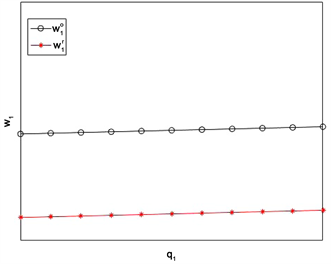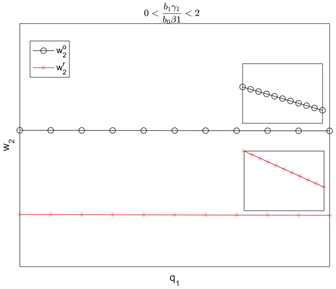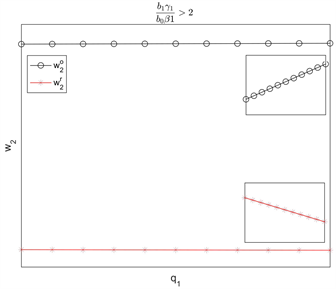Figure 2. The effect of q1 on wholesale prices. (The small image is an enlarged view of the figure)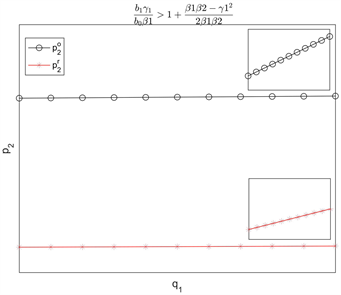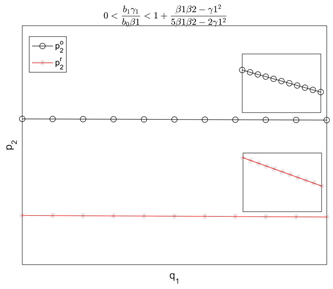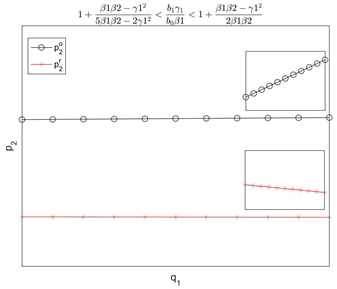Figure 3. The effect of q1 on retail prices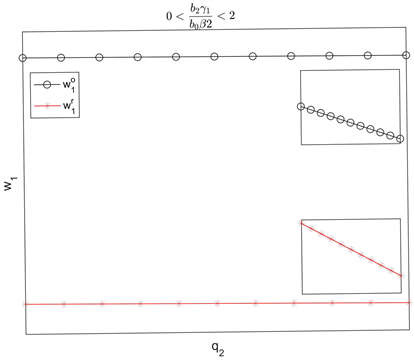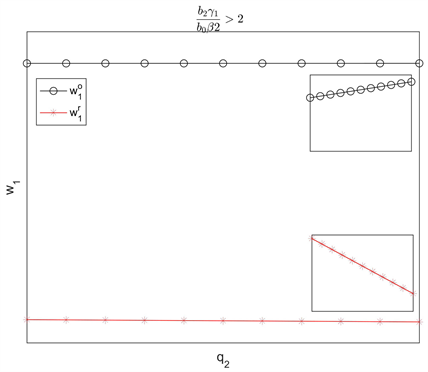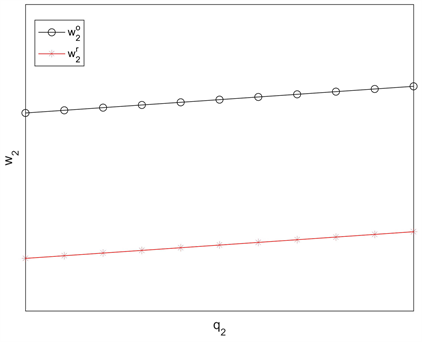Figure 4. The effect of q2 on wholesale prices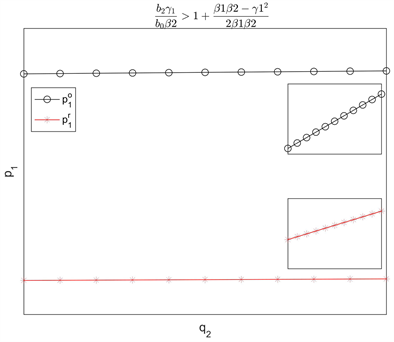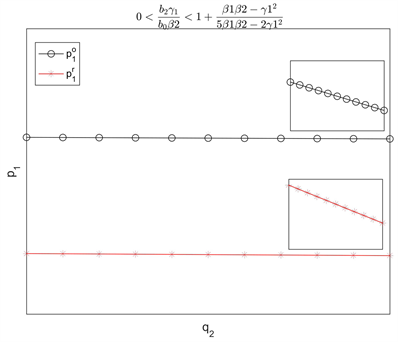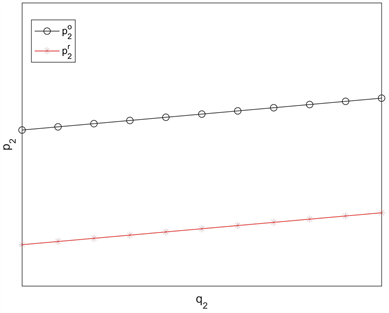Figure 5. The effect of q2 on retail prices

5. 结论与展望

${a}_{i}$ ：产品i的基本市场规模 $\left(i=1,2\right)$

${\beta }_{i}$ ：自身价格敏感系数 $\left(i=1,2\right)$

${b}_{i}$ ：自身质量水平敏感系数 $\left(i=1,2\right)$

${\gamma }_{1}$ ：两种产品之间的品牌渠道的交叉价格敏感性

${b}_{0}$ ：两种产品之间的品牌渠道的质量水平敏感性

${d}_{i}$ ：产品i的需求 $\left(i=1,2\right)$

${w}_{i}$ ：单位产品i的批发价 $\left(i=1,2\right)$

${p}_{i}$ ：单位产品i的零售价 $\left(i=1,2\right)$

${k}_{i}$ ：产品i的质量提升成本系数 $\left(i=1,2\right)$

${q}_{i}$ ：产品i的质量水平 $\left(i=1,2\right)$

${\pi }_{m1}$ ：制造商A的利润

${\pi }_{m2}$ ：制造商B的利润

${\Pi }_{1}$ ：制造商A的相对利润

${\Pi }_{2}$ ：制造商B的相对利润

NOTES

*通讯作者。

  Maiti, T. and Giri, B.C. (2017) Two-Way Product Recovery in a Closed-Loop Supply Chain with Variable Markup under Price and Quality Dependent Demand. International Journal of Production Economics, 183, 259-272. https://doi.org/10.1016/j.ijpe.2016.09.025  Li, T. and Ma, J.H. (2015) Complexity Analysis of Dual-Channel Game Model with Different Managers’ Business Objectives. Communications in Nonlinear Science and Numerical Simulation, 20, 199-208. https://doi.org/10.1016/j.cnsns.2014.05.012  Elsadany, A.A. (2017) Dynamics of a Cournot Duopoly Game with Bounded Rationality Based on Relative Profit Maximization. Applied Mathematics and Computation, 294, 253-263. https://doi.org/10.1016/j.amc.2016.09.018  Chen, J.X., et al. (2017) Price and Quality Decisions in Dual-Channel Supply Chains. European Journal of Operational Research, 259, 935-948. https://doi.org/10.1016/j.ejor.2016.11.016  Xie, Y., Tai, A.H., Ching, W.K., et al. (2016) Pricing Strategy for a Two-Echelon Supply Chain with Optimized Return Effort Level. International Journal of Production Economics, 182, 185-195. https://doi.org/10.1016/j.ijpe.2016.08.026  Giri, B.C., Chakraborty, A. and Maiti, T. (2015) Quality and Pricing Decisions in a Two-Echelon Supply Chain under Multi-Manufacturer Competition. International Journal of Advanced Manufacturing Technology, 78, 1927-1941. https://doi.org/10.1007/s00170-014-6779-2  张铁柱, 刘志勇, 滕春贤, 等. 基于二层规划的供应链定价决策研究[J]. 控制与决策, 2005, 20(9): 992-995.  Zhao, J., Hou, X., Guo, Y., et al. (2017) Pricing Policies for Complementary Products in a Dual-Channel Supply Chain. Applied Mathematical Modelling, 49, 437-451. https://doi.org/10.1016/j.apm.2017.04.023  Banker, R.D., Khosla, I. and Sinha, K.K. (1998) Quality and Competition. Management Science, 44, 1179-1192. https://doi.org/10.1287/mnsc.44.9.1179  Tsay, A.A. and Agrawal, N. (2000) Channel Dynamics under Price and Service Competition. Manufacturing and Service Operations Management, 2, 372-391. https://doi.org/10.1287/msom.2.4.372.12342  Moorthy, K.S. (1988) Product and Price Competition in a Du-opoly. Marketing Science, 7, 141-168. https://doi.org/10.1287/mksc.7.2.141  Gibbons, R. and Murphy, K.J. (1990) Relative Performance Evaluation for Chief Executive Officers. Industrial & Labor Relations Review, 43, 30S-51S. https://doi.org/10.1177/001979399004300303  Hattori, M. and Tanaka, Y. (2014) Incentive for Adoption of New Technology in Duopoly under Absolute and Relative Profit Maximization. Economics Bulletin, 34, 2051-2059.  Matsumura, T., Matsushima, N. and Cato, S. (2013) Competitionveness and R&D Competition Re-visited. Economic Modelling, 31, 541-547. https://doi.org/10.1016/j.econmod.2012.12.016  Tanaka, Y. (2013) Irrelevance of the Choice of Strategic Variables in Duopoly under Relative Profit. Economics & Business Letters, 2, 75. https://doi.org/10.17811/ebl.2.2.2013.75-83